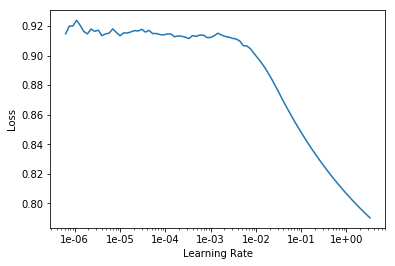# Is this learning rate finder curve normal?

Hi,

I’m having the following curve for my lr_find(), is this normal ?My task is a custom model to match question with response, at first i’m freezing the encoder, and feeding the last hidden state, avg pooling and max pooling to a two head MLP and the final representaton for both heads are fed to cosine similarity layer (scaled to be in the range of 0 to 1) followed by a binary cross entropy loss function.

======
I expect to be normal as when freezing the rnn output is all about predicting next word, so it might not be a very good feature selection to match based on, so when the learning rate is high, it’s starting to overfit somehow.

Here’s a description of the learner.
The pairwise cosine similarity is not used.

``````> SequentialRNN(
(0): MultiBatchEncoder_(
(module): AWD_LSTM(
(encoder_dp): EmbeddingDropout(
)
(rnns): ModuleList(
(0): WeightDropout(
(module): LSTM(400, 1152, batch_first=True)
)
(1): WeightDropout(
(module): LSTM(1152, 1152, batch_first=True)
)
(2): WeightDropout(
(module): LSTM(1152, 400, batch_first=True)
)
)
(input_dp): RNNDropout()
(hidden_dps): ModuleList(
(0): RNNDropout()
(1): RNNDropout()
(2): RNNDropout()
)
)
)
(q_path): Sequential(
(0): BatchNorm1d(1200, eps=1e-05, momentum=0.1, affine=True, track_running_stats=True)
(1): Dropout(p=0.1)
(2): Linear(in_features=1200, out_features=300, bias=True)
(3): ReLU(inplace)
(4): BatchNorm1d(300, eps=1e-05, momentum=0.1, affine=True, track_running_stats=True)
(5): Dropout(p=0.1)
(6): Linear(in_features=300, out_features=300, bias=True)
(7): ReLU(inplace)
)
(r_path): Sequential(
(0): BatchNorm1d(1200, eps=1e-05, momentum=0.1, affine=True, track_running_stats=True)
(1): Dropout(p=0.1)
(2): Linear(in_features=1200, out_features=300, bias=True)
(3): ReLU(inplace)
(4): BatchNorm1d(300, eps=1e-05, momentum=0.1, affine=True, track_running_stats=True)
(5): Dropout(p=0.1)
(6): Linear(in_features=300, out_features=300, bias=True)
(7): ReLU(inplace)
)
(distance): PairwiseDistance()
(cosine): CosineSimilarity()
)
)
``````